International
Tables for
Crystallography
Volume B
Reciprocal space
Edited by U. Shmueli

International Tables for Crystallography (2010). Vol. B, ch. 1.5, pp. 177-178   | 1 | 2 |

## Section 1.5.3.3. Representations of the translation groupand the reciprocal lattice

M. I. Aroyoa* and H. Wondratschekb

aDepartamento de Fisíca de la Materia Condensada, Facultad de Cienca y Technología, Universidad del País Vasco, Apartado 644, 48080 Bilbao, Spain , and bInstitut für Kristallographie, Universität, D-76128 Karlsruhe, Germany
Correspondence e-mail:  wmpararm@lg.ehu.es

#### 1.5.3.3. Representations of the translation groupand the reciprocal lattice

| top | pdf |

For representation theory we follow the terminology of BC and CDML.

Letbe referred to a primitive basis. For the following, the infinite set of translations, based on discrete cyclic groups of infinite order, will be replaced by a (very large) finite set in the usual way, assuming the Born–von Karman boundary conditionsto hold, where, (0, 1, 0) or (0, 0, 1) andis a large integer for i = 1, 2 or 3, respectively. Then for any lattice translation (I, t),holds, where Nt is the column. If the (infinitely many) translations mapped in this way onto (I, o) form a normal subgroupof, then the mapping described by (1.5.3.3)is a homomorphism. There exists a factor groupofrelative towith translation subgroupwhich is finite and is sometimes called the finite space group.

Only the irreps of these finite space groups will be considered. The definitions of space-group type, symmorphic space group etc. can be transferred to these groups. Becauseis Abelian,is also Abelian. Replacing the space groupbymeans that the especially well developed theory of representations of finite groups can be applied, cf. Lomont (1959), Jansen & Boon (1967). For convenience, the prime ′ will be omitted and the symbolwill be used instead of;will be denoted byin the following.

Because(formerly) is Abelian, its irrepsare one-dimensional and consist of (complex) roots of unity. Owing to equations (1.5.3.2)and (1.5.3.3), the irrepsofhave the formwhere t is the column,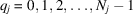,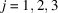, andandare integers.

Given a primitive basisof L, mathematicians and crystallographers define the basis of the dual or reciprocal latticebywhereis the scalar product between the vectors andis the unit matrix (see e.g. Chapter 1.1, Section 1.1.3). Texts on the physics of solids redefine the basisof the reciprocal lattice, lengthening each of the basis vectorsby the factor. Therefore, in the physicist's convention the relation between the bases of direct and reciprocal lattice reads (cf. BC, p. 86):In the present chapter only the physicist's basis of the reciprocal lattice is employed, and hence the use ofshould not lead to misunderstandings. The set of all vectors K,2integer, is called the lattice reciprocal to L or the reciprocal lattice.3

If one adopts the notation of IT A, Part 5, the basis of direct space is denoted by a row, wheremeans transposed. For reciprocal space, the basis is described by a column.

To each lattice generated from a basisa reciprocal lattice is generated from the basis. Both lattices, L and, can be compared most easily by referring the direct lattice L to its conventional basisas defined in Chapters 2.1and 9.1of IT A. In this case, the lattice L may be primitive or centred. Ifforms a primitive basis of L, i.e. if L is primitive, then the basisforms a primitive basis of. If L is centred, i.e.is not a primitive basis of L, then there exists a centring matrix P,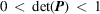, by which three linearly independent vectors of L with rational coefficients are generated from those with integer coefficients, cf. IT A, Table 5.1.3.1.

Moreover, P can be chosen such that the set of vectorsforms a primitive basis of L. Then the basis vectorsof the lattice reciprocal to the lattice generated byare determined byand form a primitive basis of.

Because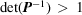, not all vectors K of the form (1.5.3.7)belong to. Ifare the (integer) coefficients of these vectors K referred toandare the vectors of, thenis a vector ofif and only if the coefficientsare integers. In other words,has to fulfil the equationAs is well known, the Bravais type of the reciprocal latticeis not necessarily the same as that of its direct lattice L. If W is the matrix of a (point-) symmetry operation of the direct lattice, referred to its basis, thenis the matrix of the same symmetry operation of the reciprocal lattice but referred to the dual basis. This does not affect the symmetry because in a (symmetry) group the inverse of each element in the group also belongs to the group. Therefore, the (point) symmetries of a lattice and its reciprocal lattice are always the same. However, there may be differences in the matrix descriptions due to the different orientations of L andrelative to the symmetry elements of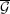and due to the reference to the different basesand. For example, if L has the point symmetry (Hermann–Mauguin symbol), then the symbol for the point symmetry ofisand vice versa.

### References

Jansen, L. & Boon, M. (1967). Theory of Finite Groups. Applications in Physics: Symmetry Groups of Quantum Mechanical Systems. Amsterdam: North-Holland.
Lomont, J. S. (1959). Applications of Finite Groups. New York: Academic Press.